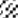# GATE 2021 Petroleum Engineering Previous Year PaperGATE 2021 Petroleum Engineering Previous Year Paper

General Aptitude (GA)

Q.1 – Q.5 Multiple Choice Question (MCQ), carry ONE mark each (for each wrong answer: – 1/3).

1. 6 – Q. 10 Multiple Choice Question (MCQ), carry TWO marks each (for each wrong answer: – 2/3).##### Petroleum Engineering (PE)

Q.1 – Q.13 Multiple Choice Question (MCQ), carry ONE mark each (for each wrong answer: – 1/3).

Q.14 – Q.19 Multiple Select Question (MSQ), carry ONE mark each (no negative marks

Q.26 – Q.37 Multiple Choice Question (MCQ), carry TWO mark each (for each wrong answer: – 2/3)

Q.38 – Q.39 Multiple Select Question (MSQ), carry TWO mark each (no negative marks).

Q.40 – Q.55 Numerical Answer Type (NAT), carry TWO mark each (no negative marks).